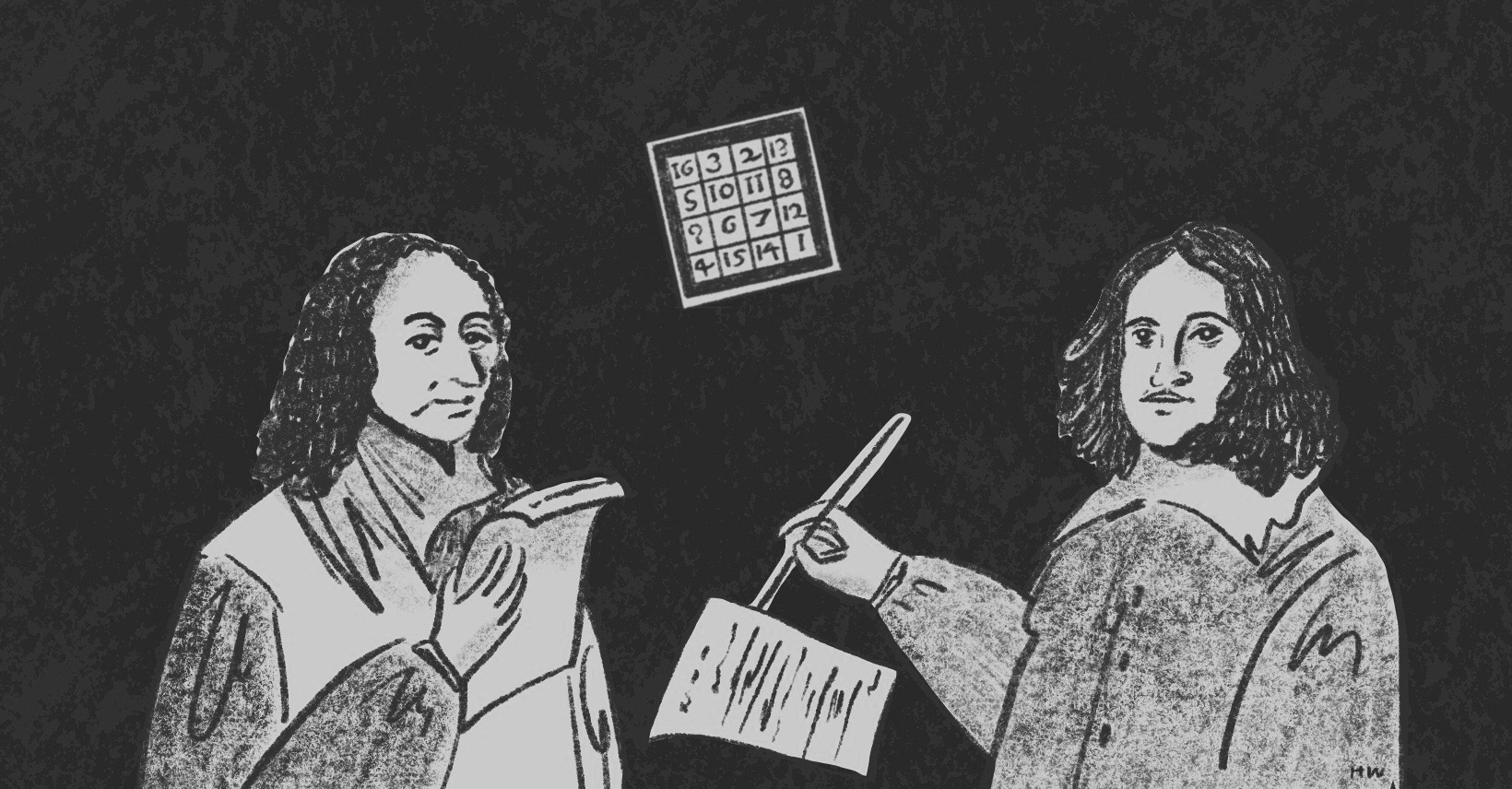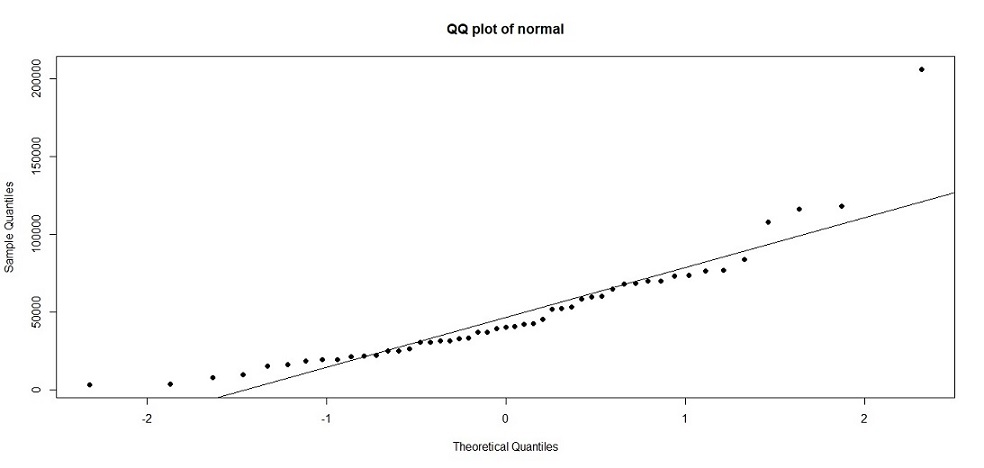×資料管理 統計分析 相關資源 巨人肩膀 語法索引 關於作者

## 簡介

• shapiro.test() -Shapiro常態分配檢定
• ks.test() -Kolmogorov-Smirnov常態分配檢定
• qqnorm() -繪製Q-Q分位圖
• qqline() -繪製Q-Q參數曲線
• t.test() -t檢定
• sample() -隨機抽樣

1. 單一樣本t檢定：適用於檢定樣本平均數與研究者設定的平均數是否有差異。

2. 獨立樣本t檢定：適用於檢定兩組獨立樣本的平均數是否有差異。

3. 成對樣本t檢定：適用於檢定兩組相依樣本的平均數是否有差異。

4. 變異數分析：適用於檢定三組以上的樣本平均數是否有差異。

## 成對樣本t檢定

$t=\frac{\overline{x}_{diff}-\mu}{s_{diff}/\sqrt{n}}$

$$\overline{x}_{diff}$$=兩個樣本差異的平均值。

$$\mu$$=常數0。

$$s_{diff}$$=兩個樣本差異的標準差。

$$n$$=樣本數。

 > hospital_population<-read.csv("c:/Users/USER/downloads/nhi_service.csv", header=T, sep=",") #讀取資料 > hospital_population[25, "Before_201806"]<-99 #處理遺漏值 > hospital_population[37, "Before_201806"]<-99 #處理遺漏值 > hospital_population$Before_201806[hospital_population$Before_201806==99]<-NA #處理遺漏值 > hospital_sample<-hospital_population[sample(nrow(hospital_population),50),] #從母體中隨機抽取50個樣本 > dim(hospital_sample) #顯示樣本數，確定抽樣結果  50 5 > attach(hospital_sample) > shapiro.test(Before_201806) #常態分配測試，樣本數<50執行Shapiro-Wilk test Shapiro-Wilk normality test data: Before_201806 W = 0.84161, p-value = 1.1e-05 > ks.test(Before_201806, "pnorm") #常態分配測試，樣本數>50執行Kolmogorov-Smirnov test One-sample Kolmogorov-Smirnov test data: Before_201806 D = 1, p-value = 3.331e-16 alternative hypothesis: two-sided 

 > qqnorm(Before_201806, main="QQ plot of normal", pch=19) #繪製Q-Q plot > qqline(Before_201806) #繪製趨勢線> t.test(Before_201806, After_201906, paired=TRUE, na.action="na.exclude") Paired t-test data: Before_201806 and After_201906 t = 2.4666, df = 48, p-value = 0.01726 alternative hypothesis: true difference in means is not equal to 0 95 percent confidence interval: 272.9296 2680.1725 sample estimates: mean of the differences 1476.551 

 > mean(Before_201806, na.rm=T)  48506.69 > mean(After_201906, na.rm=T)  46561.88 

 > mean(hospital_population$Before_201806, na.rm=T)  57723.95 > mean(hospital_population$After_201906, na.rm=T)  55732.47 

## 獨立樣本t檢定

$t=\frac{\overline{x}_{1}-\overline{x}_{2}}{\sqrt{\frac{s_{1}^{2}}{n_{1}}+\frac{s_{2}^{2}}{n_{2}}}}$

$$\overline{x}_{1}, \overline{x}_{2}$$=第一組樣本、第二組樣本的平均數。

$$s_{1}^{2}, s_{2}^{2}$$=第一組樣本、第二組樣本的標準差。

$$n_{1}, n_{2}$$=第一組樣本、第二組樣本的樣本數。

 > trad_med<-read.csv("c:/Users/USER/downloads/gender_traditionalmed.csv", header=T, sep=",") #載入資料 > dim(trad_med)  22 3 > attach(trad_med) > shapiro.test(Male) #常態分配測試，樣本數<50執行Shapiro-Wilk test > shapiro.test(Female) #常態分配測試，樣本數<50執行Shapiro-Wilk test 

Shapiro-Wilk test檢測樣本常態分配後可發現男性p值>.05，符合常態分配假設；女性p值<.05，不符合常態分配假設。接下來使用F檢定來看看兩組樣本的母體變異數是否有差異：

 > var.test(Male, Female) #檢測變異數是否相等 F test to compare two variances data: Male and Female F = 0.16033, num df = 21, denom df = 21, p-value = 9.514e-05 alternative hypothesis: true ratio of variances is not equal to 1 95 percent confidence interval: 0.06656604 0.38616977 sample estimates: ratio of variances 0.1603303 

F test的p值<.05，拒絕虛無假設，母體變異數不相等。稍後的t檢定必須套用變異數不相等的假設。

 > t.test(Male, Female, var.equal=FALSE) Welch Two Sample t-test data: Male and Female t = -17.556, df = 27.565, p-value < 2.2e-16 alternative hypothesis: true difference in means is not equal to 0 95 percent confidence interval: -1170724.3 -925922.3 sample estimates: mean of x mean of y 2700413 3748736 

t檢定p值小於.05，拒絕虛無假設，男女的平均就醫人數有顯著差異。從平均就醫人數來看，男生2700413人，女生3748736人，女生看中醫的次數確實比男生多。

## 單一樣本t檢定

$t=\frac{\overline{x}-\mu}{s/\sqrt{n}}$

$$\overline{x}$$=樣本平均數。

$$\mu$$=母體平均數或理論值。

$$s$$=樣本標準差$$\sqrt{\frac{\sum_{i=1}^{n}(x_{i}-\overline{x})^2}{n-1}}$$

$$n$$=樣本數。

 > year<-c(2012,2013,2014,2015,2016,2017,2018,2019,2020,2021) > temperature<-c(14.9, 15.6, 16.2, 15.8, 15.8, 17.4, 16.6, 17.6, 16.8, 15.0) > temp_Jan<-data.frame(year, temperature) > shapiro.test(temp_Jan$temperature) Shapiro-Wilk normality test data: temp_Jan$temperature W = 0.95122, p-value = 0.6829 

Shapiro test樣本符合常態分配，接下來作t檢定。

 > t.test(temp_Jan$temperature, mu=15.4) One Sample t-test data: temp_Jan$temperature t = 2.6289, df = 9, p-value = 0.02741 alternative hypothesis: true mean is not equal to 15.4 95 percent confidence interval: 15.50742 16.83258 sample estimates: mean of x 16.17